## Example Questions

← Previous 1 3 4 5 6 7 8 9 18 19

### Example Question #1 : Solid Geometry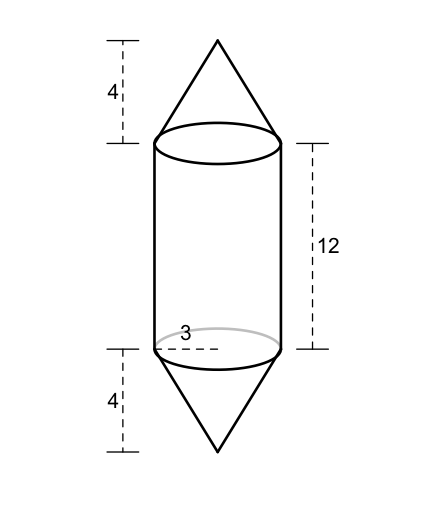The above image is a silo made from two right circular cones, and a right circular cylinder where the dimensions are given in feet. Of the following answers, which one best represents the volume of the entire silo in cubic feet?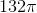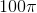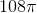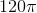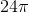Explanation:

To solve this, we need to break it down into three parts.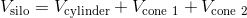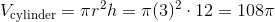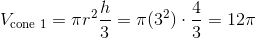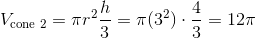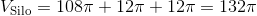### Example Question #1 : How To Find The Length Of An Edge Of A Cube

The number of square units in the surface area of a cube is twice as large as the number of cubic units in its volume. What is the cube's volume, in cubic units?

216

36

9

27

108

27

Explanation:

The number of square units in the surface area of a cube is given by the formula 6s2, where s is the length of the side of the cube in units. Moreover, the number of cubic units in a cube's volume is equal to s3

Since the number of square units in the surface area is twice as large as the cubic units of the volume, we can write the following equation to solve for s:

6s2 = 2s3

Subtract 6s2 from both sides.

2s3 – 6s2 = 0

Factor out 2s2 from both terms.

2s2(s – 3) = 0

We must set each factor equal to zero.

2s2 = 0, only if s = 0; however, no cube has a side length of zero, so s can't be zero.

Set the other factor, s – 3, equal to zero.

s – 3 = 0

s = 3

This means that the side length of the cube is 3 units. The volume, which we previously stated was equal to s3, must then be 33, or 27 cubic units.

### Example Question #1 : Cubes

You own a Rubik's cube with a volume of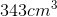. What is the edge length of the cube?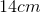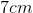No enough information to solve.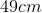Explanation:

You own a Rubik's cube with a volume of. What is the edge length of the cube?

To solve for edge length, think of the volume of a cube formula: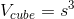Now, we have the volume, so just rearrange it to solve for side length: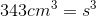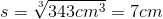### Example Question #2 : Cubes

If a cube is 3” on all sides, what is the length of the diagonal of the cube?

9

3√2

4√3

27

3√3

3√3

Explanation:

General formula for the diagonal of a cube if each side of the cube = s

Use Pythagorean Theorem to get the diagonal across the base:

s2 + s2 = h2

And again use Pythagorean Theorem to get cube’s diagonal, then solve for d:

h2 + s2 = d2

s2 + s2 + s2 = d2

3 * s2 = d2

d = √ (3 * s2) = s √3

So, if s = 3 then the answer is 3√3

### Example Question #1 : How To Find The Diagonal Of A Cube

A cube is inscribed in a sphere of radius 1 such that all 8 vertices of the cube are on the surface of the sphere.  What is the length of the diagonal of the cube?

(2)

1

8

2

(3)

2

Explanation:

Since the diagonal of the cube is a line segment that goes through the center of the cube (and also the circumscribed sphere), it is clear that the diagonal of the cube is also the diameter of the sphere.  Since the radius = 1, the diameter = 2.

### Example Question #3 : How To Find The Diagonal Of A Cube

What is the length of the diagonal of a cube with volume of 512 in3?

2√(6) in

4√(3) in

8√(3) in

8 in

8√(3) in

Explanation:

The first thing necessary is to determine the dimensions of the cube.  This can be done using the volume formula for cubes: V = s3, where s is the length of the cube. For our data, this is:

s3 = 512, or (taking the cube root of both sides), s = 8.

The distance from corner to corner of the cube will be equal to the distance between (0,0,0) and (8,8,8).  The distance formula for three dimensions is very similar to that of 2 dimensions (and hence like the Pythagorean Theorem):

d√( (x1 – x2)2 + (y1 – y2)2  + (z1 – z2)2

Or for our simpler case:

d = √( (x)2 + (y)2  + (z)2) = √( (s)2 + (s)2  + (s)2) = √( (8)2 + (8)2  + (8)2) = √( 64 + 64 + 64) = √(64 * 3) = 8√(3)

### Example Question #4 : How To Find The Diagonal Of A Cube

What is the length of the diagonal of a cube with volume of 1728 in3?

3√(3) in

12√(3) in

18 in

12 in

6√(3) in

12√(3) in

Explanation:

The first thing necessary is to determine the dimensions of the cube.  This can be done using the volume formula for cubes: V = s3, where s is the length of the cube. For our data, this is:

s3 = 1728, or (taking the cube root of both sides), s = 12.

The distance from corner to corner of the cube will be equal to the distance between (0,0,0) and (12,12,12).  The distance formula for three dimensions is very similar to that of 2 dimensions (and hence like the Pythagorean theorem):

d = √( (x1 – x2)2 + (y1 – y2)2  + (z1 – z2)2

Or, for our simpler case:

d = √( (x)2 + (y)2  + (z)2) = √( (s)2 + (s)2  + (s)2) = √( (12)2 + (12)2  + (12)2) = √( 144 + 144 + 144) = √(3 * 144) = 12√(3) = 12√(3)

### Example Question #5 : How To Find The Diagonal Of A Cube

What is the length of the diagonal of a cube with surface area of 294 in2?

21√(2)

14

21

7√(3)

7√(3)

Explanation:

The first thing necessary is to determine the dimensions of the cube. This can be done using the surface area formula for cubes: A = 6s2, where s is the length of the cube. For our data, this is:

6s2 = 294

s2 = 49

(taking the square root of both sides) s = 7

The distance from corner to corner of the cube will be equal to the distance between (0,0,0) and (7,7,7). The distance formula for three dimensions is very similar to that of 2 dimensions (and hence like the Pythagorean Theorem):

d = √((x1 – x2)2 + (y1 – y2)2  + (z1 – z2)2

Or for our simpler case:

d = √((x)2 + (y)2  + (z)2) = √( (s)2 + (s)2  + (s)2) = √( (7)2 + (7)2  + (7)2) = √( 49 + 49 + 49) =  √(49 * 3) = 7√(3)

### Example Question #3 : Cubes

A rectangular prism has a volume of 144 and a surface area of 192. If the shortest edge is 3, what is the length of the longest diagonal through the prism?Explanation:

The volume of a rectangular prism is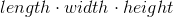.

We are told that the shortest edge is 3.  Let us call this the height.

We now have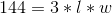, or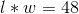.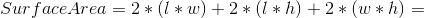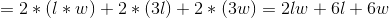Now we replace variables by known values: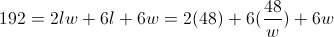Now we have: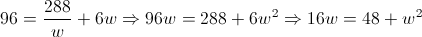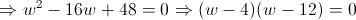We have thus determined that the other two edges of the rectangular prism will be 4 and 12.  We now need to find the longest diagonal.  This is equal to: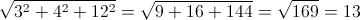If you do not remember how to find this directly, you can also do it in steps.  You first find the diagonal across one of the sides (in the plane), by using the Pythagorean Theorem.  For example, we choose the side with edges 3 and 4.  This diagonal will be: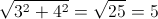We then use a plane with one side given by the diagonal we just found (length 5) and the other given by the distance of the 3rd edge (length 12).

This diagonal is then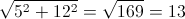.

### Example Question #1 : How To Find The Surface Area Of A Cube

What is the surface area of a cube with a volume of 1728 in3?

432 in2

864 in2

144 in2

72 in2

1728 in2

864 in2

Explanation:

This problem is relatively simple. We know that the volume of a cube is equal to s3, where s is the length of a given side of the cube. Therefore, to find our dimensions, we merely have to solve s3 = 1728. Taking the cubed root, we get s = 12.

Since the sides of a cube are all the same, the surface area of the cube is equal to 6 times the area of one face. For our dimensions, one face has an area of 12 * 12 or 144 in2. Therefore, the total surface area is 6 * 144 = 864 in2.

← Previous 1 3 4 5 6 7 8 9 18 19

### All SAT Math Resources